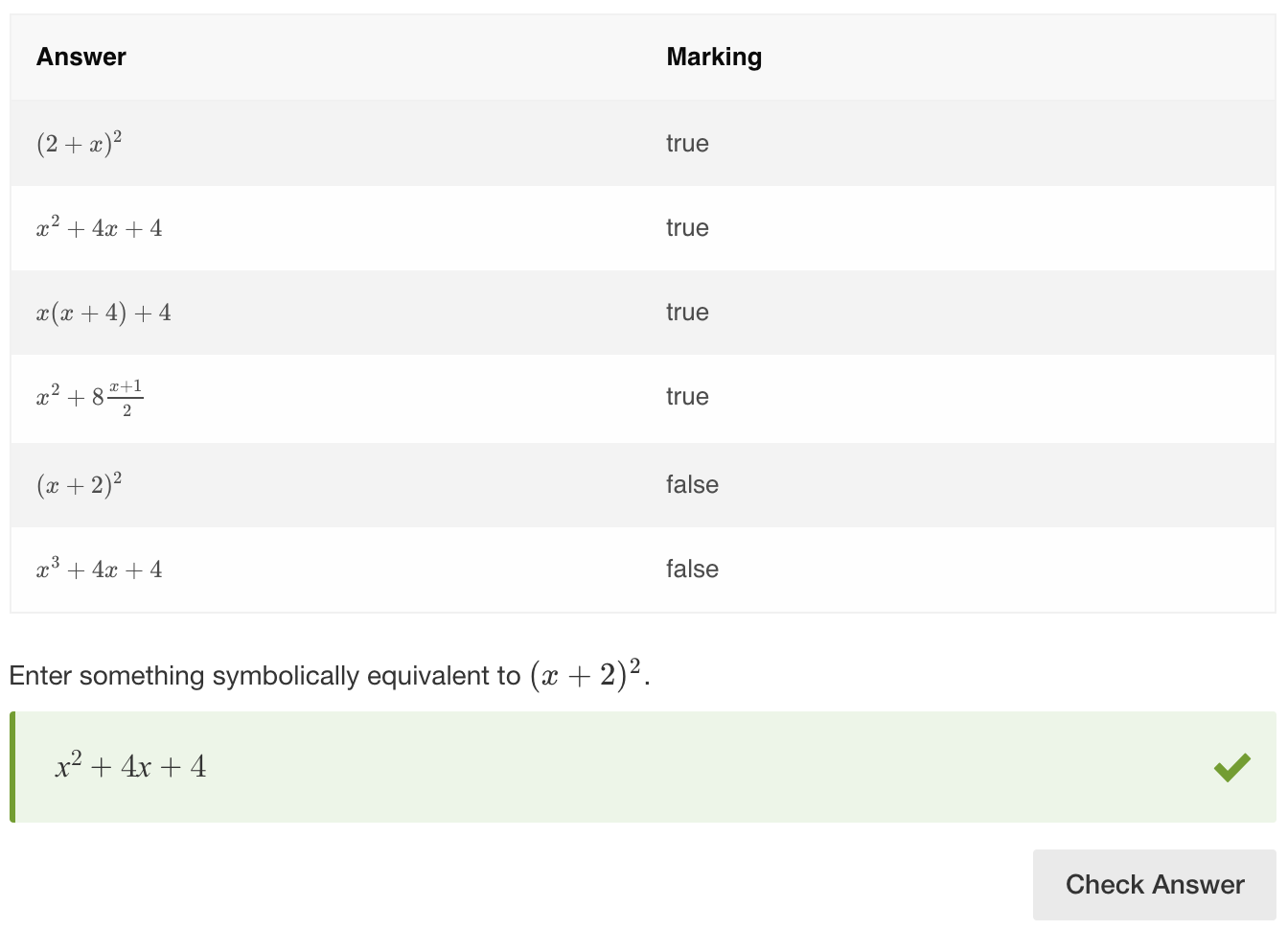# equivSymbolic

## Overview

The equivSymbolic method checks that the value entered by the learner is mathematically equivalent to the value that the Author has set in the validation, even if they are in different forms.

Use equivSymbolic when working with expressions where order or form is not important. For example, if the task is to multiply 3 and 2, any response that is mathematically equal to 6 will be considered as correct, such as 6/1, 12/2, 3+3, 6.0.

equivSymbolic accepts a wide range of inputs including decimals, fractions, variables, percentages, trigonometric, logarithmic, and exponential expressions.

• Significant decimal places
This option specifies the number of significant decimal places used to compare the learner's response and the Author's validation value. The maximum and default value is 10.
• Allow interval
This option must be enabled when the learner is expected to insert interval notation in the response area, ie  ‘[1, 4)’. Without this option enabled, the response may not validate correctly.
• Ignore text
This refers to LaTeX text only, and when enabled the scoring engine will ignore any LaTeX text the learner enters in the response area.
• Compare sides
With this option enabled, when comparing two equations the math engine will compare the corresponding sides of each equation rather than the whole equations.
• Treat 'e' as Euler's number
This option treats the variable 'e' as Euler's number. This overrides the default interpretation of 'e' as scientific notation.
• Allow decimal marks
Authors can specify what separators can be used by the learner. From the Thousand Separator drop-down dot, comma, and/or space can be selected. From the Decimal Separator drop-down either a dot or a comma can be selected. Note that the specified thousand separators and decimal separator cannot be the same, e.g. both dot.
• Treat all letters as variables
When this option is enabled, the math engine will treat all letters as variables. This is useful in cases where you want to use letters as variables that the math engine may normally recognize as units of measurement. Here are the units of measurement we support: List of S.I and US Customary Units

## Example 1 - simple use case for equivSymbolic

In this Question, the students enter a value in the response box in order to complete the equation.

Condition: anything that is equal to 100 must be entered in the response box.

#### Authoring instructions

To author this Question:

1. Click the plus icon in the authoring interface to create a new Item, choose the "Math" category, and choose "Cloze Math" from the tile view of Question types.
2. In the "Formula template" field, delete the placeholder text to the left of the Response box. To the right of the Response box, type the stimulus text label, as "= 100".
3. In the "Set correct answer(s)" section, select "Method" as "equivSymbolic" and "Value" as "100".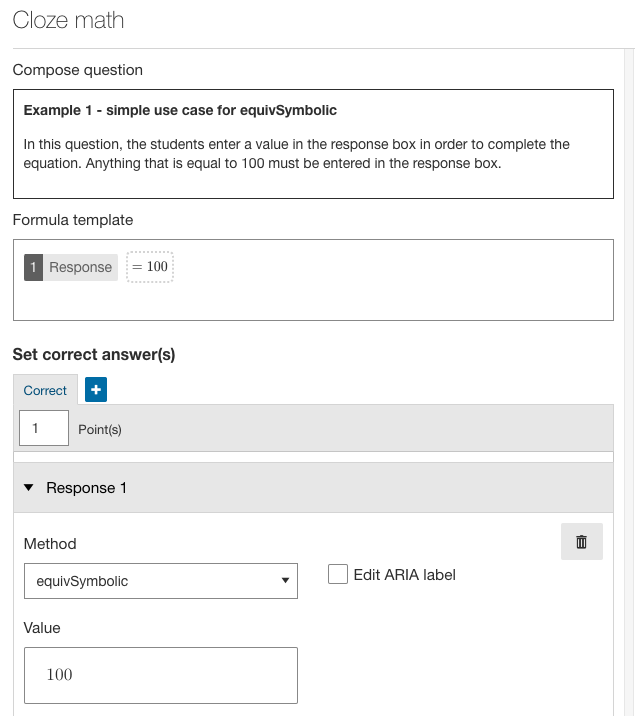Screenshot 1: authoring choices for example 1 - simple use case for equivSymbolic

#### Response table for example 1 - simple use case for equivSymbolic

Here's a range of answers that will be marked as correct with the above configuration.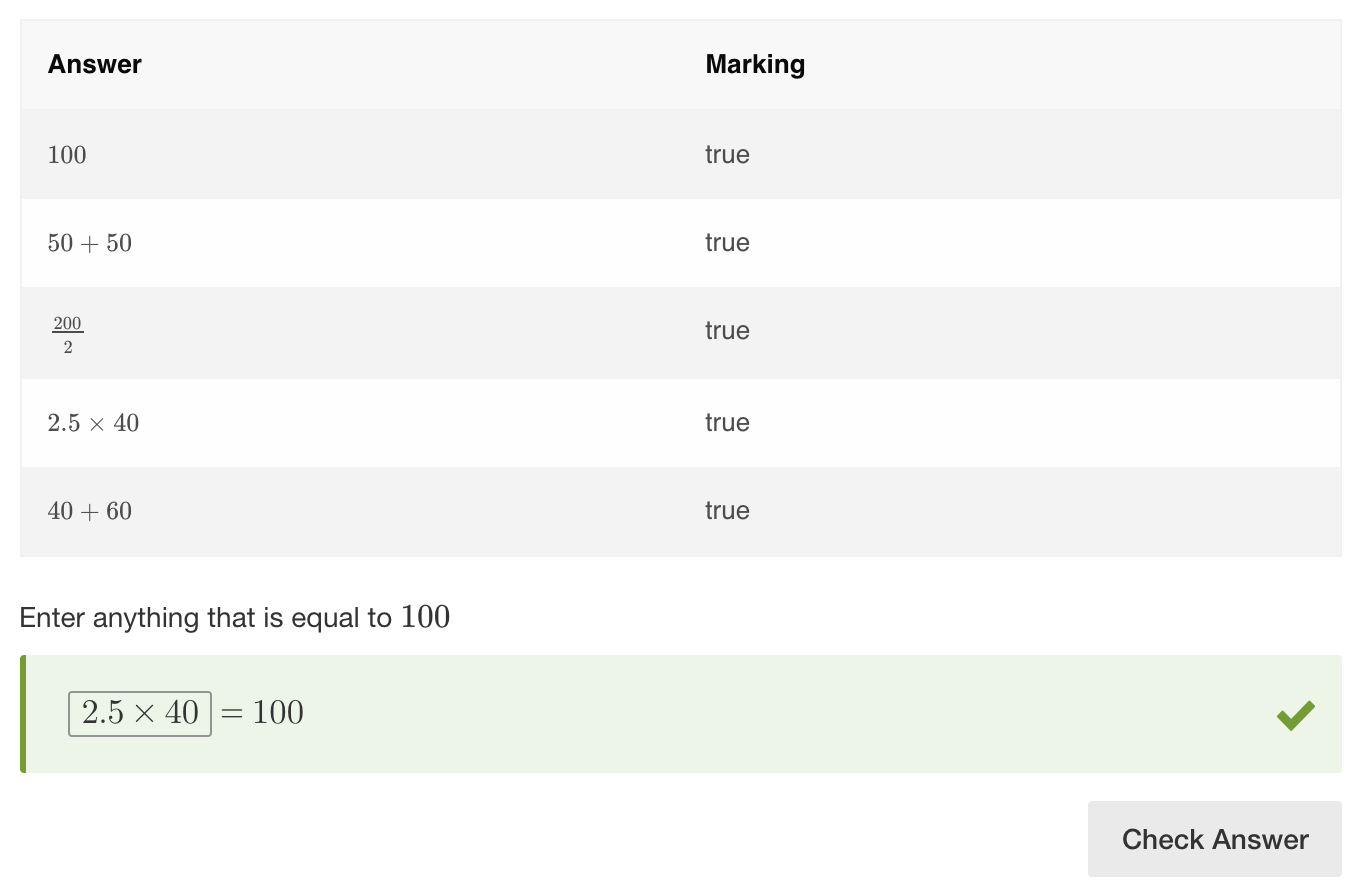Screenshot 2: response table for example 1 - simple use case for equivSymbolic

## Example 2 - equivSymbolic with algebraic expressions

In the example below, an expression in any form that is mathematically equivalent to the one in the stimulus will be correct.

Condition: enter something symbolically equivalent to (x + 2)2 (squared).

#### Authoring instructions

To author this Question:

1. Click the plus icon in the authoring interface to create a new Item, choose the "Math" category, and choose "Cloze Math" from the tile view of Question types.
2. In the "Formula template" field, delete the placeholder text to the left of the Response box.
3. In the "Set correct answer(s)" section, select "Method" as "equivSymbolic" and "Value" as "(x + 2)2(squared)".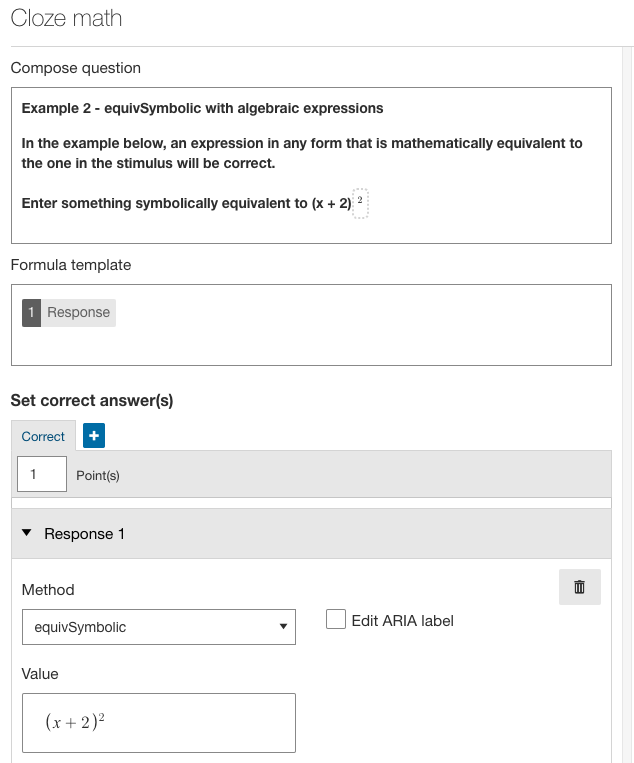Screenshot 3: authoring choices for example 2 - equivSymbolic with algebraic expressions

Response table for example 2 - equivSymbolic with algebraic expressions

Here's a range of answers that will be marked as correct with the above configuration.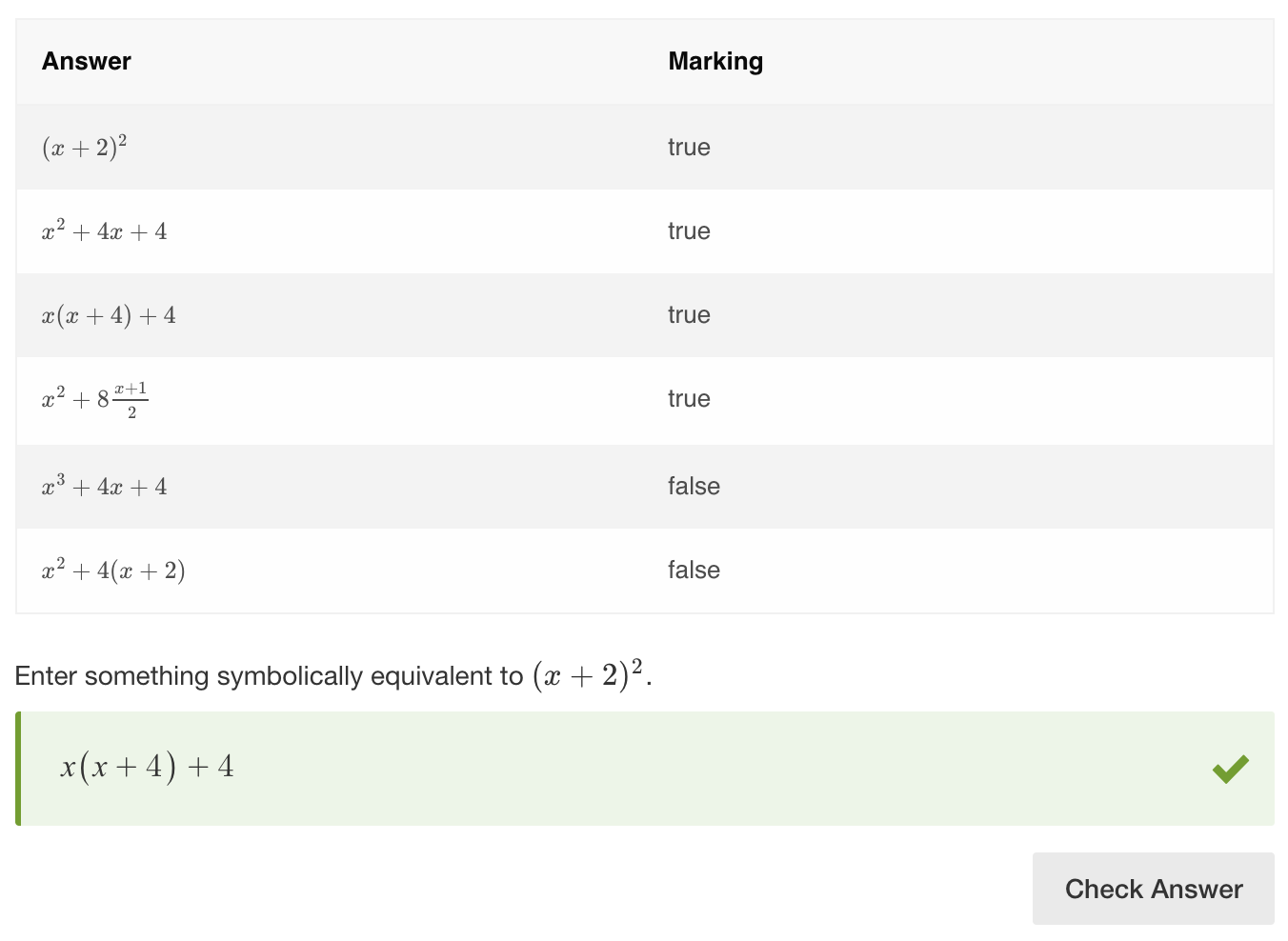Screenshot 4: response table for example 2 - equivSymbolic with algebraic expressions

## Example 3 - decimal rounding

The decimal places field is used to specify a number of significant decimal places in the response. In this example, the significant decimal places option is set to 2. This way, the learner will not need to enter all the digits after the decimal point for the response to be deemed correct.

Condition: enter anything symbolically equivalent to ⅓.

#### Authoring instructions

To author this Question:

1. Click the plus icon in the authoring interface to create a new Item, choose the "Math" category, and choose "Cloze Math" from the tile view of Question types.
2. In the "Set correct answer(s)" section, select "Method" as "equivSymbolic" and enter "Value" as "".
3. In the "Significant decimal places" field, enter "2".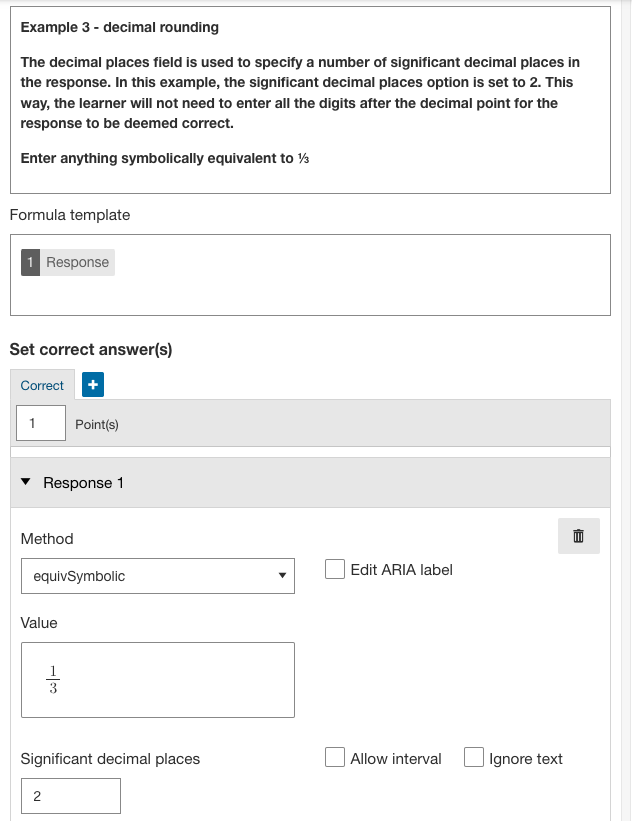Screenshot 5: authoring choices for example 3 - decimal rounding

#### Response table for example 3 - decimal rounding

Here's a range of answers that will be marked as correct with the above configuration.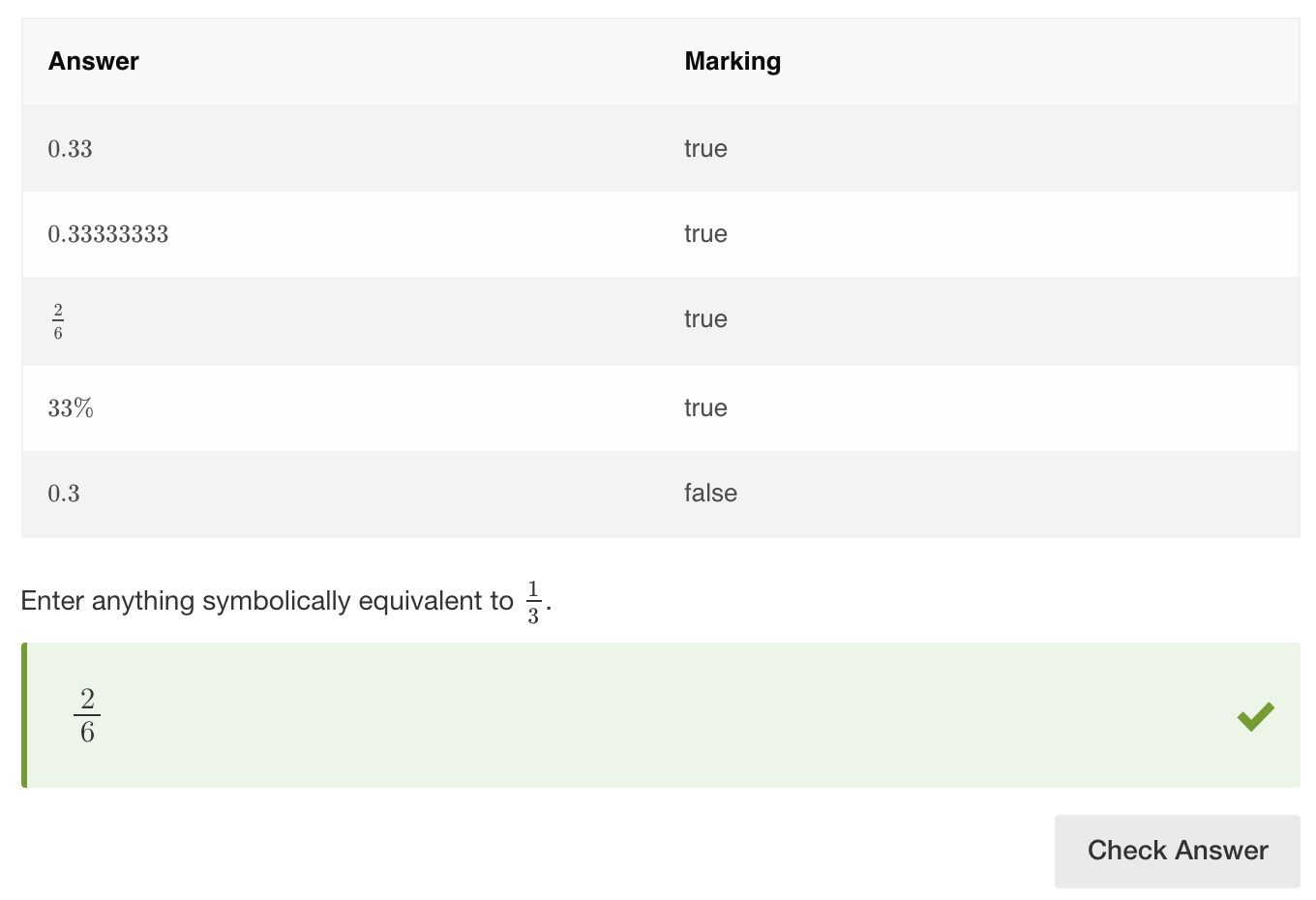Screenshot 6: response table for example 3 - decimal rounding

## Example 4 - using text blocks

You can set custom units such as ft, kg, mi, etc. as text blocks in the More options section. This means that the units will not be rendered as LaTeX.

Condition: enter anything symbolically equivalent to 100ft.

#### Authoring instructions

To author this Question:

1. Click the plus icon in the authoring interface to create a new Item, choose the "Math" category, and choose "Cloze Math" from the tile view of Question types.
2. In the "Formula template" field, delete the placeholder text to the left of the Response box.
3. In the "Set correct answer(s)", Response 1 section, select "Method" as "equivSymbolic" and click in the Value field. In the formula keypad, enter the "Value" as "100" then open the drop-down menu and select the "lb" option for "US Customary Units", and then click "ft" for feet.
4. Below the keypad configuration section, in the "text blocks" section, click the small blue "+ADD" button. This will add a text block. In the text block entry field, type "ft" for feet.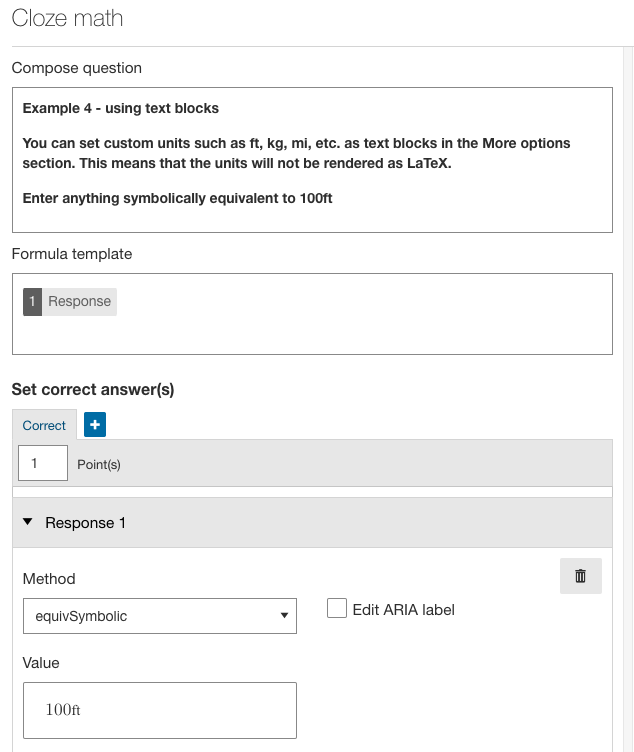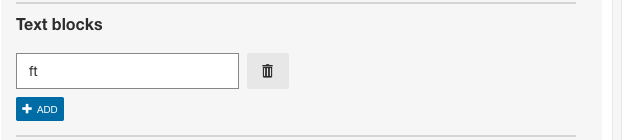Screenshot 7: authoring choices for example 4 - using text blocks

#### Response table for example 4 - using text blocks

Here's a range of answers that will be marked as correct with the above configuration.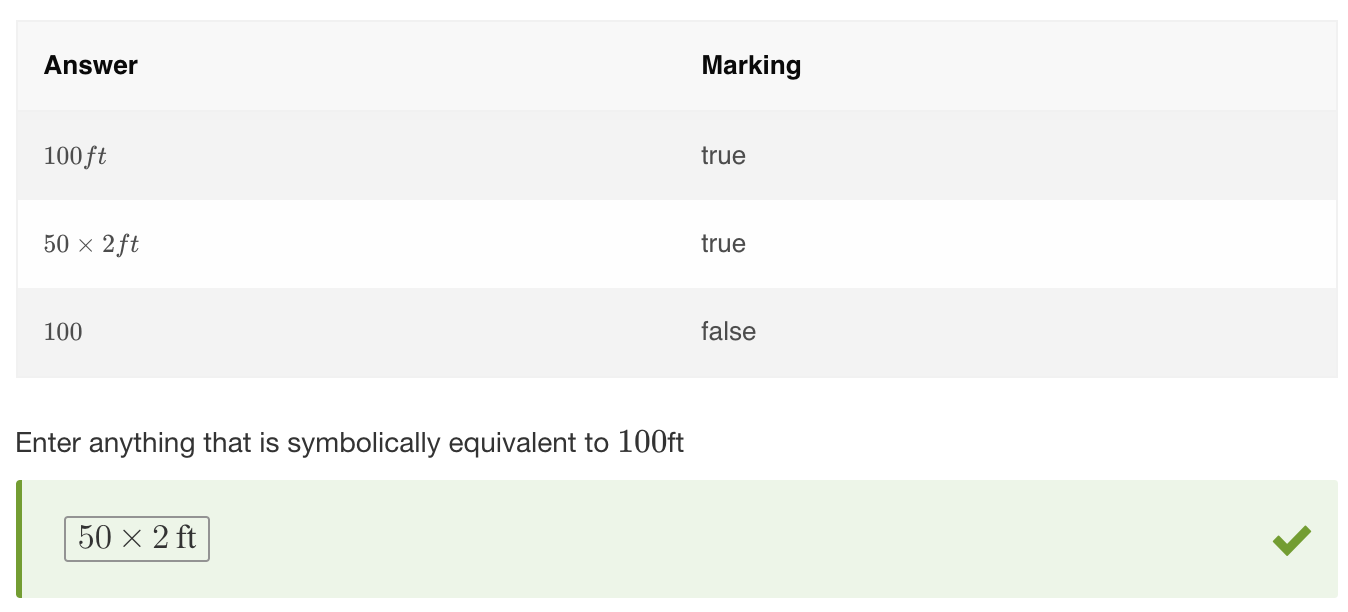## Example 5 - using compare sides

Compare sides is used with open-ended questions. It ensures the equation values entered by the student give the same mathematical result and are symbolically equivalent to the equation set by the author.

Condition: the learner will need to enter values into three entry boxes to fill out all the variables in an equation. The stimulus is like so: Alex had 4 apples, Ben gave her 3 more apples. How many apples has she now?

#### Authoring instructions

To author this Question:

1. Click the plus icon in the authoring interface to create a new Item, choose the "Math" category, and choose "Cloze Math" from the tile view of Question types.
2. In the "Formula template" field, delete the placeholder text to the left of the Response box 1.
3. In the "Set correct answer(s)", Response 1 section, select "Method" as "equivSymbolic" and click in the Value field. In the formula keypad, enter the "Value" as "4 + 3 = 7".
4. Tick the "compare sides" box. (this compares the values to the left and the right of the equals sign in the formula template)
5. Click the arrow to expand the "More options" window. In the "Layout > Response container (global) > Formula template" field, click in the field to show the formula keypad. Click the "r" box at the top right of the formula keypad window to add additional response boxes, and enter the following: "response container" + "response container" = "response container". This sets up the three entry zones for learners to fill in.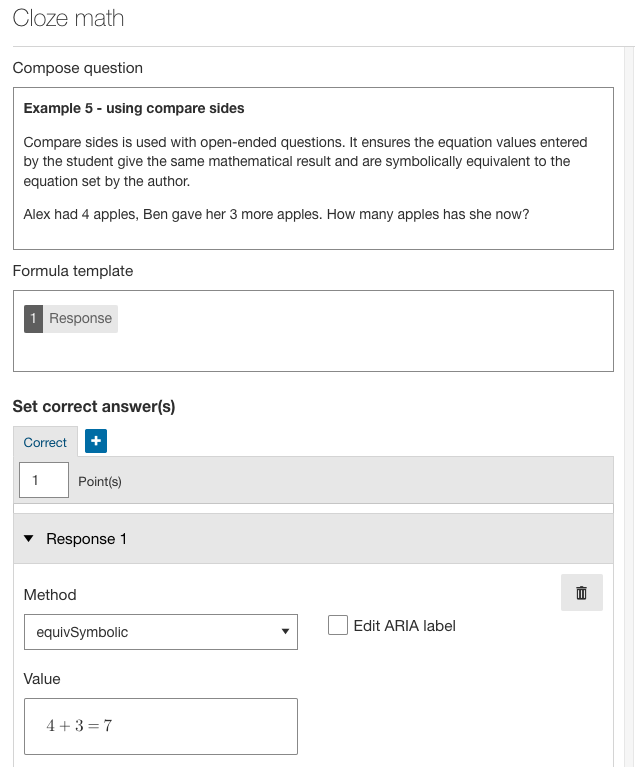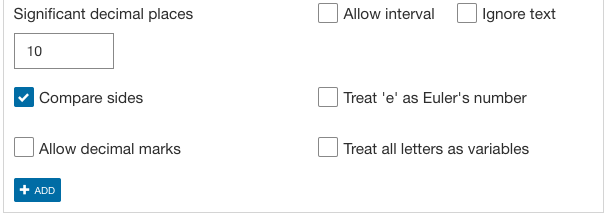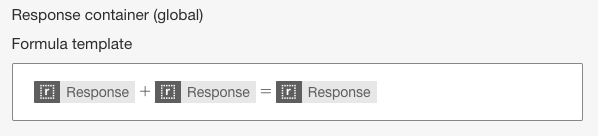Screenshot 9: authoring choices for example 5 - using compare sides

#### Response table: example 5 - using compare sides

Here's a range of answers that will be marked as correct with the above configuration.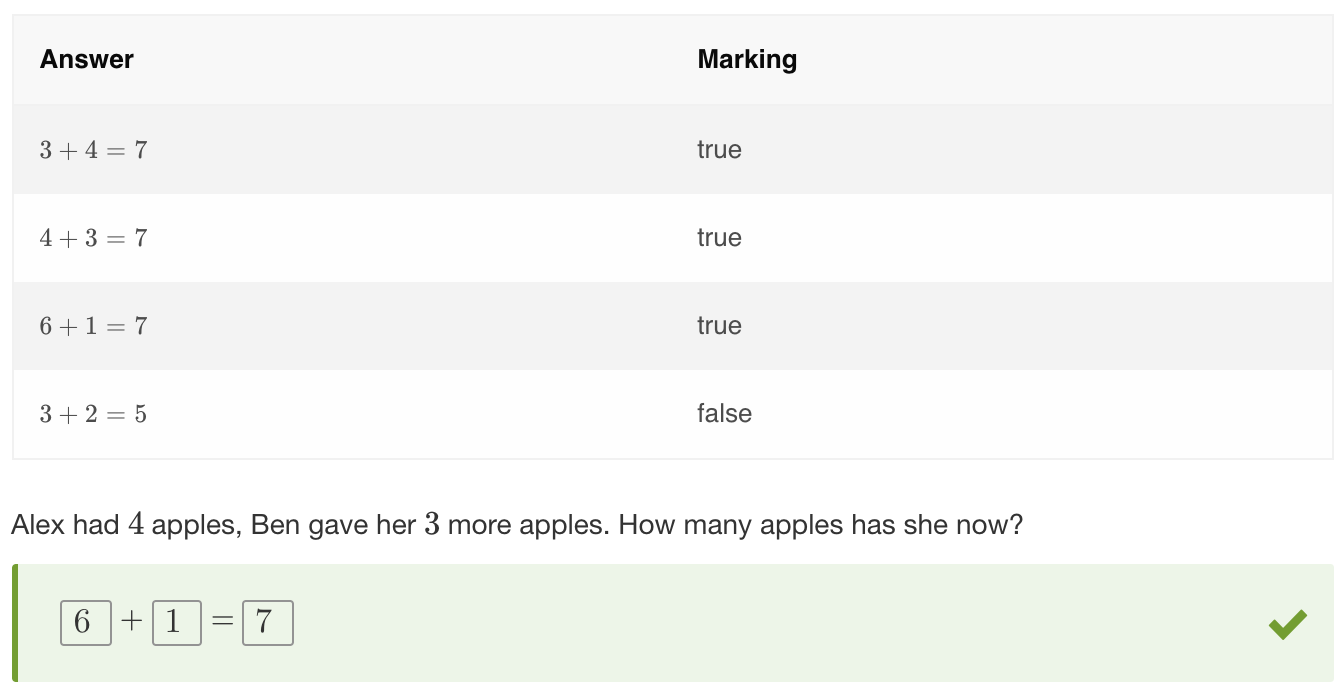## Example 6 - excluding possible correct responses

equivSymbolic is a very flexible method and is often used in conjunction with other methods.

In some cases the students might be asked to give a correct response other than the one already specified in the stimulus. Authors can set such exceptions by combining equivSymbolic and equivLiteral, then enabling the inverse result option underneath equivLiteral. Now, any symbolically equivalent equation will be correct except for the actual valid response specified in question validation.

Condition: enter something symbolically equivalent to (x + 2)2(squared).

#### Authoring instructions

To author this Question:

1. Click the plus icon in the authoring interface to create a new Item, choose the "Math" category, and choose "Cloze Math" from the tile view of Question types.
2. In the "Formula template" field, delete the placeholder text to the left of the Response box 1.
3. In the "Set correct answer(s)", Response 1 section, select "Method" as "equivSymbolic" and click in the Value field. Enter the "Value" as "(x + 2)2(squared)".
4. Click the small blue "+ADD" button, to get another response block.
5. In the newly added section, select "Method" as "equivLiteral" and click in the Value field. Enter the "Value" as "(x + 2)2(squared)".
6. Tick the "Inverse result" option.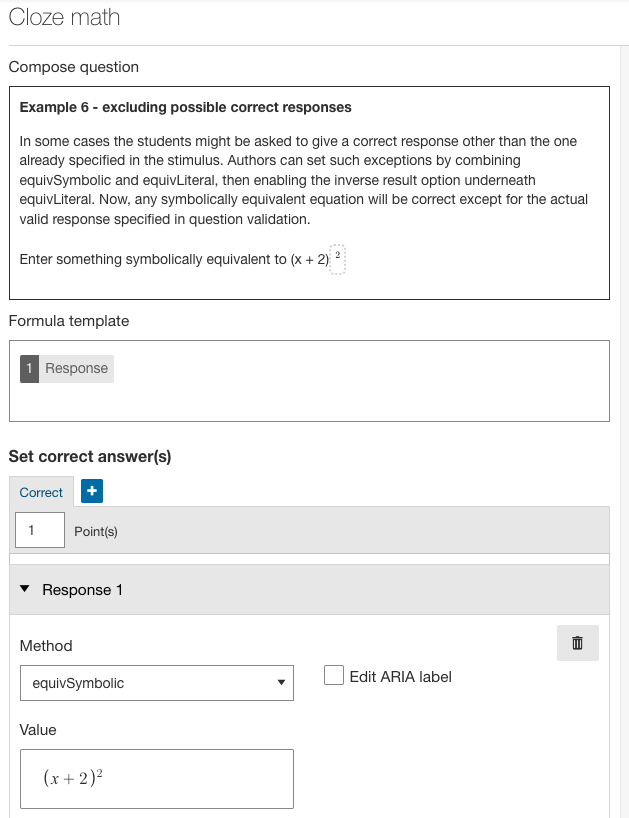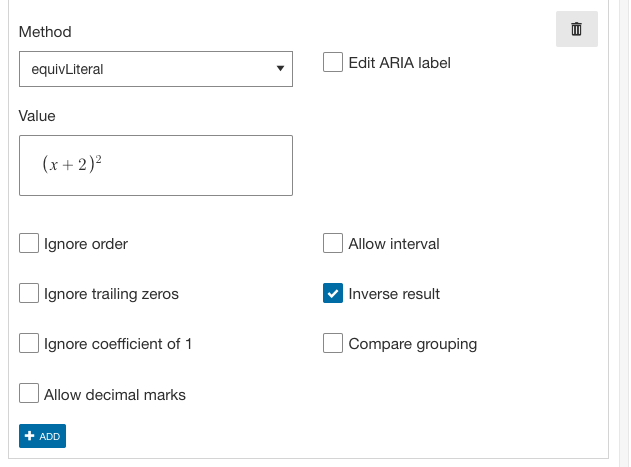Screenshot 11: authoring choices for example 6 - excluding possible correct responses

#### Response table: example 6 - excluding possible correct responses

Here's a range of answers that will be marked as correct with the above configuration. Notice that the exact example given in the stimulus is not allowed as an answer.